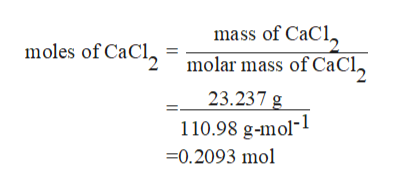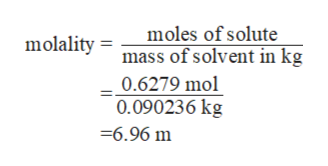# Water has a molal freezing point constant of 1.86 degrees celcius kg/mol. Calculate the freezing point of 23.237 grams of calcium chloride(CaCl2) in 90.236 grams of water. Assume complete dissociation.

Question
3 views

Water has a molal freezing point constant of 1.86 degrees celcius kg/mol. Calculate the freezing point of 23.237 grams of calcium chloride(CaCl2) in 90.236 grams of water. Assume complete dissociation.

check_circle

Step 1

Amount of Calcium chloride is 23.237 g

Amount of water is 90.236 g

Molal freezing point constant for water is 1.86 degrees celsius kg/mol.

The molar mass of Calcium chloride is 110.98 g/mol

Moles of Calcium chloride can be calculated as :help_outlineImage Transcriptionclosemass of CaCl molar mass of CaCl 23.237 g 110.98 g-mol-1 moles of CaCl 0.2093 mol fullscreen
Step 2

Calcium chloride dissociates in the following way as follows:

When  1 mol of calcium chloride breaks it forms 1 mol of calcium ion and 2 mol of chloride ions as shown in above reaction.

Thus total 3 moles are obtained by one mole of calcium chloride.

Since Calcium chloride is solute, its moles can be calculated as follows:

Moles of Calcium chloride = 0.2093×3

=0.6279 mol

Thus the moles of solute is 0.6279 mol

Step 3

Also given that,

Water is a solvent and its mass is 90.236 g or 0.090236 kg

Now mola...help_outlineImage Transcriptionclosemoles of solute mass of solvent in kg 0.6279 mol 0.090236 kg molality -6.96 m fullscreen

### Want to see the full answer?

See Solution

#### Want to see this answer and more?

Solutions are written by subject experts who are available 24/7. Questions are typically answered within 1 hour.*

See Solution
*Response times may vary by subject and question.
Tagged in

### Chemistry